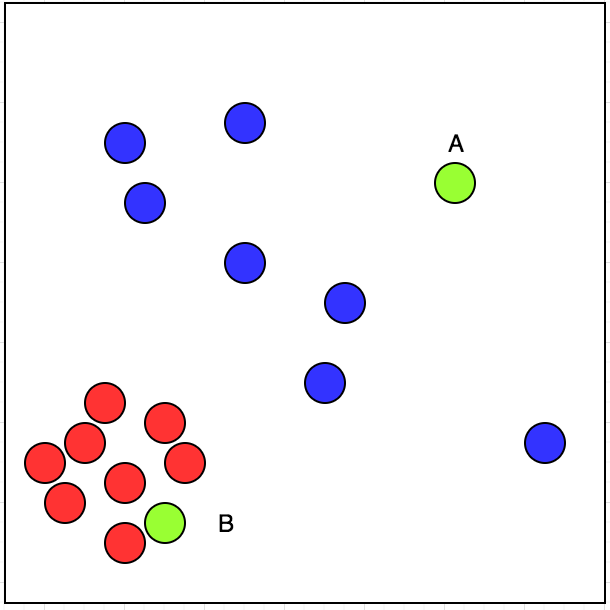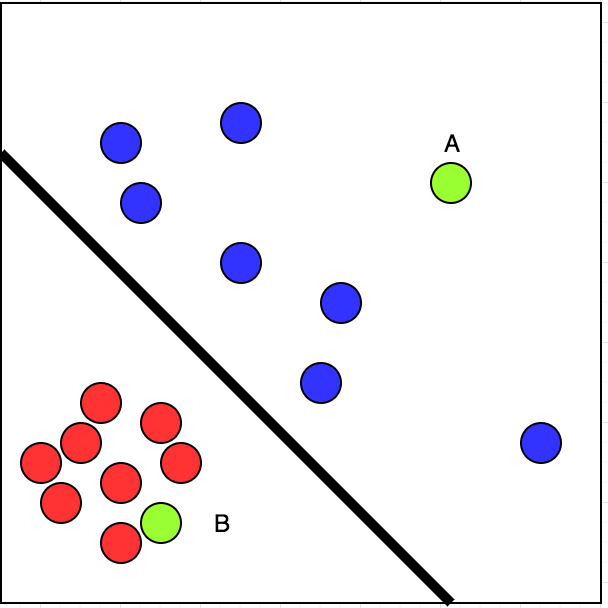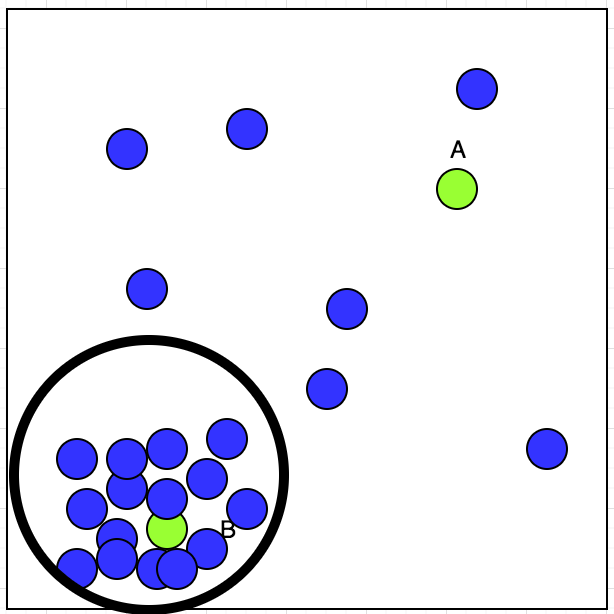Machine Learning is a kind of buzz words these days; sort of a mysterious new guy in the class whom everyone wants to be friend with.

On the onset, I would like to think Machine Learning as a part of tool kit of every Software Engineer. And conceptually it’s not different from the Mathematics, Algorithm, Probability and Statistics courses that we did in our engineering courses.

## Where is the use case for Machine Learning?

• Where we have a pattern
• Where we can’t have a mathematical formula
• Where we have a data

To summarise the three points mentioned above, wherever we know that data has a pattern but we can’t pin point the exact mathematical formula to represent the pattern; Machine Learning comes to our rescue.

However, we can apply machine learning to the problems where there is no pattern. We would not know the quality of result but how bad it can be; it was already random, right?

We can also apply machine learning to a problem where we have a mathematical problem. It’s kind of overkill but yes, machine learning will work.

But the data! We can’t do any kind of machine learning without the data. Data is a must for machine learning.

## Types of Machine Learning

• Supervised Learning
• Unsupervised Learning
• Reinforced Learning

Learning has three kind mentioned above. What’s the difference? Supervised Learning has input and correct output. For example: We have the data if a person liked the movie or not. On the basis of interviewing people and gathering their response if they liked the movie or not, we are going to predict if the movie is going to be hit or not.Let’s look at the picture above. I have visited the restaurants marked by red circle. The restaurants which I have not visited is marked by blue circle.

Now, If I have two restaurants to choose from, A and B, marked by green colour, which one will I choose?

Simple. We can classify the given data linearly into two parts. That means, we can draw a line segregating red and blue circle. Look at the picture below:Now, we can say with some confidence that chances of my visiting B is more than A. This is a case of supervised learning.

Unsupervised learning has inputs. Let’s suppose we have a taxi driver who has an option of accepting or rejecting the bookings. We have plotted his accepted booking location on map with blue circle and is shown below:Now, Taxi driver has got two bookings A and B; Which one he will accept? If we observe the plot, we can see that his accepted booking shows a cluster at lower left corner. That can be shown in the picture below:We can now say with some confidence that B has got higher chance of getting the booking accepted by the driver.

Reinforced Learning has input, output(not necessarily the correct one) and the grade of output. Reinforced learning finds its application in playing games where it rates each moves and then learn accordingly.

Read further post in this series:

Perceptron in Machine Learning: First learning model

How do machines learn?